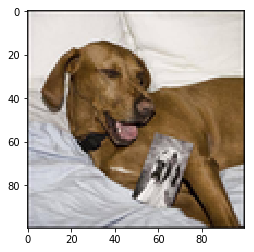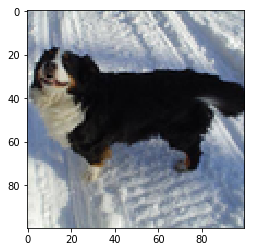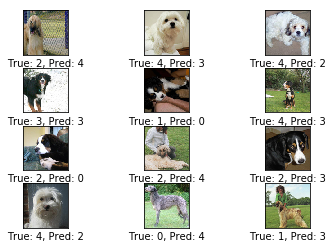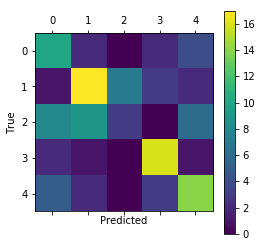# Kaggle Dog Breeds – Image recognition

My main code for image recognition :

In :
```%matplotlib inline
import matplotlib.pyplot as plt
import tensorflow as tf
import numpy as np
import time
from datetime import timedelta
import math
import os
import scipy.misc
from scipy.stats import itemfreq
from random import sample
import pickle
from sklearn.metrics import confusion_matrix
from sklearn.model_selection import train_test_split

# Image manipulation.
import PIL.Image
from IPython.display import display
from resizeimage import resizeimage

#Panda for csv
import pandas as pd

#Open a Zip File
from zipfile import ZipFile
from io import BytesIO

tf.__version__
```
Out:
`'1.4.0'`
In :
```#We unzip the train and test zip file
archive_train = ZipFile("Data/train.zip", 'r')
archive_test = ZipFile("Data/test.zip", 'r')

#This line shows the 5 first image name of the train database
archive_train.namelist()[0:5]

#This line shows the number of images in the train database
len(archive_train.namelist()[:])-1 #we must remove the 1st value
```
Out:
`10222`
In :
```# This function help to create  a pickle file gathering all the image from a zip folder
###############
def DataBase_creator(archivezip, nwigth, nheight, save_name):
#We choose the archive (zip file) + the new wigth and height for all the image which will be reshaped

# Start-time used for printing time-usage below.
start_time = time.time()

s = (len(archivezip.namelist()[:])-1, nwigth, nheight,3) #10000 = 100 x 100 number of features because image is 100x100 pixels
allImage = np.zeros(s)

for i in range(1,len(archivezip.namelist()[:])):
image = PIL.Image.open(filename) # open colour image
image = PIL.Image.open(filename) # open colour image
image = resizeimage.resize_cover(image, [nwigth, nheight])
image = np.array(image)
image = np.clip(image/255.0, 0.0, 1.0) #255 = max of the value of a pixel

allImage[i-1]=image

#we save the newly created data base
pickle.dump(allImage, open( save_name + '.p', "wb" ) )

# Ending time.
end_time = time.time()

# Difference between start and end-times.
time_dif = end_time - start_time

# Print the time-usage.
print("Time usage: " + str(timedelta(seconds=int(round(time_dif)))))
```
In :
```DataBase_creator(archivezip = archive_train, nwigth = 100, nheight = 100 , save_name = "train")
```
```Time usage: 0:01:53
```
In :
```DataBase_creator(archivezip = archive_test, nwigth = 100, nheight = 100 , save_name = "test")
```
```Time usage: 0:01:37
```
In [ ]:
```######Loading Train and Test DataBase re-written + train_id
```
In :
```#load TRAIN
train = pickle.load( open( "train.p", "rb" ) )
train.shape
```
Out:
`(10222, 100, 100, 3)`
In :
```#load TEST
test = pickle.load( open( "test.p", "rb" ) )
test.shape
```
Out:
`(10357, 90, 90, 3)`
In :
```lum_img = train[100,:,:,:]
plt.imshow(lum_img)
plt.show()
```In [ ]:
```################
#### FILTER TRAIN AND LABEL DATASET###########
################
```
In :
```#######Upload the zip (input data base)########
labels_raw[0:5]
```
Out:
id breed
0 000bec180eb18c7604dcecc8fe0dba07 boston_bull
1 001513dfcb2ffafc82cccf4d8bbaba97 dingo
2 001cdf01b096e06d78e9e5112d419397 pekinese
3 00214f311d5d2247d5dfe4fe24b2303d bluetick
4 0021f9ceb3235effd7fcde7f7538ed62 golden_retriever
In :
```#######Get the N most represented breeds########
def main_breeds(labels_raw, Nber_breeds , all_breeds='TRUE'):
labels_freq_pd = itemfreq(labels_raw["breed"])
labels_freq_pd = labels_freq_pd[labels_freq_pd[:, 1].argsort()[::-1]] #[::-1] ==> to sort in descending order

if all_breeds == 'FALSE':
main_labels = labels_freq_pd[:,0][0:Nber_breeds]
else:
main_labels = labels_freq_pd[:,0][:]

labels_raw_np = labels_raw["breed"].as_matrix() #transform in numpy
labels_raw_np = labels_raw_np.reshape(labels_raw_np.shape,1)

labels_filtered_index = np.where(labels_raw_np == main_labels)

return labels_filtered_index

labels_filtered_index = main_breeds(labels_raw = labels_raw, Nber_breeds = 5, all_breeds='FALSE')
labels_filtered_index.shape
```
Out:
`(588,)`
In :
```labels_filtered = labels_raw.iloc[labels_filtered_index,:]
train_filtered = train[labels_filtered_index,:,:,:]
train_filtered.shape
```
Out:
`(588, 100, 100, 3)`
In :
```lum_img = train_filtered[90,:,:,:]
plt.imshow(lum_img)
plt.show()
```In [ ]:
```######LABELS######
```
In :
```#We select the labels from the N main breeds
labels = labels_filtered["breed"].as_matrix()
labels = labels.reshape(labels.shape,1) #labels.shape looks faster than using len(labels)
labels.shape
```
Out:
`(588, 1)`
In :
```def matrix_Bin(labels):
labels_bin=np.array([])

labels_name, labels0 = np.unique(labels, return_inverse=True)
labels0

for _, i in enumerate(itemfreq(labels0)[:,0].astype(int)):
labels_bin0 = np.where(labels0 == itemfreq(labels0)[:,0][i], 1., 0.)
labels_bin0 = labels_bin0.reshape(1,labels_bin0.shape)

if (labels_bin.shape == 0):
labels_bin = labels_bin0
else:
labels_bin = np.concatenate((labels_bin,labels_bin0 ),axis=0)

print("Nber SubVariables {0}".format(itemfreq(labels0)[:,0].shape))
labels_bin = labels_bin.transpose()
print("Shape : {0}".format(labels_bin.shape))

return labels_name, labels_bin
```
In :
```labels_name, labels_bin = matrix_Bin(labels = labels)
labels_bin[0:2]
```
```Nber SubVariables 5
Shape : (588, 5)
```
Out:
```array([[ 0.,  0.,  0.,  0.,  1.],
[ 0.,  0.,  0.,  1.,  0.]])```
In :
```#You can proceed backward with np.argmax to find the breed of an image
labels_cls = np.argmax(labels_bin, axis=1)
labels_name[labels_cls]
#labels_cls
```
Out:
`'scottish_deerhound'`
In [ ]:
```######Creation of the Train DataBase and Test DataBase
```
In :
```num_test = 0.20
X_train, X_test, y_train, y_test = train_test_split(train_filtered, labels_bin, test_size=num_test, random_state=23)
X_train.shape
```
Out:
`(470, 100, 100, 3)`
In :
```#Creation of the Train DataBase and Test DataBase
#x% of the observations will belong to the Train DataBase

def train_test_creation(x, data, toPred):
indices = sample(range(data.shape),int(x * data.shape))
indices = np.sort(indices, axis=None)
index = np.arange(data.shape)
reverse_index = np.delete(index, indices,0)

train_toUse = data[indices]
train_toPred = toPred[indices]
test_toUse = data[reverse_index]
test_toPred = toPred[reverse_index]

return train_toUse, train_toPred, test_toUse, test_toPred

df_train_toUse, df_train_toPred, df_test_toUse, df_test_toPred = train_test_creation(0.7, train_filtered, labels_bin)
df_train_toUse.shape
```
Out:
`(411, 100, 100, 3)`
In :
```df_test_toPred_cls = np.argmax(y_test, axis=1)
df_test_toPred_cls[0:9]
```
Out:
`array([3, 3, 4, 4, 0, 2, 1, 2, 1])`
In [ ]:
```######TENSORFLOW
```
In :
```# Our images are 100 pixels in each dimension.
img_size = 100

# Number of colour channels for the images: 3
num_channels = 3

# Images are stored in one-dimensional arrays of this length.
img_size_flat = img_size * img_size

# Image Shape
img_shape = (img_size, img_size, num_channels)

# Number of classes : 5 breeds
num_classes = 5
```
In :
```def new_weights(shape):
return tf.Variable(tf.truncated_normal(shape, stddev=0.05))
#outputs random value from a truncated normal distribution

def new_biases(length):
return tf.Variable(tf.constant(0.05, shape=[length]))
#outputs the constant value 0.05
```
In :
```def new_conv_layer(input,              # The previous layer.
num_input_channels, # Num. channels in prev. layer.
filter_size,        # Width and height of each filter.
num_filters,        # Number of filters.
use_pooling=True):  # Use 2x2 max-pooling.

# Shape of the filter-weights for the convolution.
# This format is determined by the TensorFlow API.
shape = [filter_size, filter_size, num_input_channels, num_filters]

# Create new weights aka. filters with the given shape.
weights = new_weights(shape=shape)

# Create new biases, one for each filter.
biases = new_biases(length=num_filters)

# Create the TensorFlow operation for convolution.
# Note the strides are set to 1 in all dimensions.
# The first and last stride must always be 1,
# because the first is for the image-number and
# the last is for the input-channel.
# But e.g. strides=[1, 2, 2, 1] would mean that the filter
# is moved 2 pixels across the x- and y-axis of the image.
# The padding is set to 'SAME' which means the input image
# is padded with zeroes so the size of the output is the same.
layer = tf.nn.conv2d(input=input,
filter=weights,
strides=[1, 1, 1, 1],

# Add the biases to the results of the convolution.
# A bias-value is added to each filter-channel.
layer += biases

# Use pooling to down-sample the image resolution?
if use_pooling:
# This is 2x2 max-pooling, which means that we
# consider 2x2 windows and select the largest value
# in each window. Then we move 2 pixels to the next window.
layer = tf.nn.max_pool(value=layer,
ksize=[1, 2, 2, 1],
strides=[1, 2, 2, 1],

# Rectified Linear Unit (ReLU).
# It calculates max(x, 0) for each input pixel x.
# This adds some non-linearity to the formula and allows us
layer = tf.nn.relu(layer)

# Note that ReLU is normally executed before the pooling,
# but since relu(max_pool(x)) == max_pool(relu(x)) we can
# save 75% of the relu-operations by max-pooling first.

# We return both the resulting layer and the filter-weights
# because we will plot the weights later.
return layer, weights
```
In :
```def flatten_layer(layer):
# Get the shape of the input layer.
layer_shape = layer.get_shape()

# The shape of the input layer is assumed to be:
# layer_shape == [num_images, img_height, img_width, num_channels]

# The number of features is: img_height * img_width * num_channels
# We can use a function from TensorFlow to calculate this.
num_features = layer_shape[1:4].num_elements()

# Reshape the layer to [num_images, num_features].
# Note that we just set the size of the second dimension
# to num_features and the size of the first dimension to -1
# which means the size in that dimension is calculated
# so the total size of the tensor is unchanged from the reshaping.
layer_flat = tf.reshape(layer, [-1, num_features])

# The shape of the flattened layer is now:
# [num_images, img_height * img_width * num_channels]

# Return both the flattened layer and the number of features.
return layer_flat, num_features
```
In :
```def new_fc_layer(input,          # The previous layer.
num_inputs,     # Num. inputs from prev. layer.
num_outputs,    # Num. outputs.
use_relu=True): # Use Rectified Linear Unit (ReLU)?

# Create new weights and biases.
weights = new_weights(shape=[num_inputs, num_outputs])
biases = new_biases(length=num_outputs)

# Calculate the layer as the matrix multiplication of
# the input and weights, and then add the bias-values.
layer = tf.matmul(input, weights) + biases

# Use ReLU?
if use_relu:
layer = tf.nn.relu(layer)

return layer
```
In :
```x = tf.placeholder(tf.float32, shape=[None, img_size, img_size, num_channels], name='x')
x_image = tf.reshape(x, [-1, img_size, img_size, num_channels]) #-1 put everything as 1 array
y_true = tf.placeholder(tf.float32, shape=[None, num_classes], name='y_true')
y_true_cls = tf.argmax(y_true, dimension=1)
```
```WARNING:tensorflow:From <ipython-input-34-1a9086e61b77>:4: calling argmax (from tensorflow.python.ops.math_ops) with dimension is deprecated and will be removed in a future version.
Instructions for updating:
```
In :
```# Convolutional Layer 1.
filter_size1 = 5          # Convolution filters are 5 x 5 pixels.
num_filters1 = 8         # There are 8 of these filters.

# Convolutional Layer 2.
filter_size2 = 5          # Convolution filters are 5 x 5 pixels.
num_filters2 = 16      # There are 16 of these filters.

# Fully-connected layer.
fc_size = 128
```
In :
```layer_conv1, weights_conv1 = \
new_conv_layer(input=x_image,
num_input_channels=num_channels,
filter_size=filter_size1,
num_filters=num_filters1,
use_pooling=False)

layer_conv2, weights_conv2 = \
new_conv_layer(input=layer_conv1,
num_input_channels=num_filters1,
filter_size=filter_size2,
num_filters=num_filters2,
use_pooling=True)
```
In :
```layer_flat, num_features = flatten_layer(layer_conv2)
```
In :
```layer_fc1 = new_fc_layer(input=layer_flat,
num_inputs=num_features,
num_outputs=fc_size,
use_relu=True)

layer_fc1

layer_fc2 = new_fc_layer(input=layer_fc1,
num_inputs=fc_size,
num_outputs=num_classes,
use_relu=False)

layer_fc2
```
Out:
`<tf.Tensor 'add_3:0' shape=(?, 5) dtype=float32>`
In :
```y_pred = tf.nn.softmax(layer_fc2)
y_pred_cls = tf.argmax(y_pred, dimension=1)
```
In :
```cross_entropy = tf.nn.softmax_cross_entropy_with_logits(logits=layer_fc2,
labels=y_true)
cost = tf.reduce_mean(cross_entropy)

correct_prediction = tf.equal(y_pred_cls, y_true_cls)
accuracy = tf.reduce_mean(tf.cast(correct_prediction, tf.float32))
```
In :
```session = tf.Session()

def init_variables():
session.run(tf.global_variables_initializer())
```
In :
```batch_size = 25

#function next_batch
def next_batch(num, data, labels):
'''
Return a total of `num` random samples and labels.
'''
idx = np.arange(0 , len(data))
np.random.shuffle(idx)
idx = idx[:num]
data_shuffle = [data[i] for i in idx]
labels_shuffle = [labels[i] for i in idx]

return np.asarray(data_shuffle), np.asarray(labels_shuffle)
```
In :
```def optimize(num_iterations, X):
global total_iterations

start_time = time.time()

for i in range(num_iterations):
total_iterations += 1
# Get a batch of training examples.
# x_batch now holds a batch of images and
# y_true_batch are the true labels for those images.
x_batch, y_true_batch = next_batch(batch_size, X_train, y_train)

# Put the batch into a dict with the proper names
# for placeholder variables in the TensorFlow graph.
feed_dict_train = {x: x_batch,
y_true: y_true_batch}
feed_dict_test = {x: X_test,
y_true: y_test}

# Run the optimizer using this batch of training data.
# TensorFlow assigns the variables in feed_dict_train
# to the placeholder variables and then runs the optimizer.
session.run(optimizer, feed_dict=feed_dict_train)

# Print status every X iterations.
if (total_iterations % X == 0) or (i ==(num_iterations -1)):
# Calculate the accuracy on the training-set.
acc_train = session.run(accuracy, feed_dict=feed_dict_train)
acc_test = session.run(accuracy, feed_dict=feed_dict_test)

msg = "Iteration: {0:>6}, Training Accuracy: {1:>6.1%}, Test Accuracy: {2:>6.1%}"
print(msg.format(total_iterations, acc_train, acc_test))

# Ending time.
end_time = time.time()

# Difference between start and end-times.
time_dif = end_time - start_time

# Print the time-usage.
print("Time usage: " + str(timedelta(seconds=int(round(time_dif)))))
```
In :
```init_variables()
total_iterations = 0
```
In :
```optimize(num_iterations=500, X=50)
```
```Iteration:     50, Training Accuracy:  44.0%, Test Accuracy:  24.6%
Iteration:    100, Training Accuracy:  56.0%, Test Accuracy:  34.7%
Iteration:    150, Training Accuracy:  52.0%, Test Accuracy:  39.8%
Iteration:    200, Training Accuracy:  64.0%, Test Accuracy:  37.3%
Iteration:    250, Training Accuracy:  64.0%, Test Accuracy:  48.3%
Iteration:    300, Training Accuracy:  92.0%, Test Accuracy:  40.7%
Iteration:    350, Training Accuracy:  80.0%, Test Accuracy:  44.9%
Iteration:    400, Training Accuracy:  92.0%, Test Accuracy:  39.8%
Iteration:    450, Training Accuracy:  96.0%, Test Accuracy:  40.7%
Iteration:    500, Training Accuracy:  96.0%, Test Accuracy:  50.8%
Time usage: 0:04:43
```
In :
```def plot_images(images, cls_true, cls_pred=None):
assert len(images) == len(cls_true) == 12

# Create figure with 3x3 sub-plots.
fig, axes = plt.subplots(4, 3)

for i, ax in enumerate(axes.flat):
# Plot image.
ax.imshow(images[i].reshape(img_shape), cmap='binary')

# Show true and predicted classes.
if cls_pred is None:
xlabel = "True: {0}".format(cls_true[i])
else:
xlabel = "True: {0}, Pred: {1}".format(cls_true[i], cls_pred[i])

# Show the classes as the label on the x-axis.
ax.set_xlabel(xlabel)

# Remove ticks from the plot.
ax.set_xticks([])
ax.set_yticks([])

# Ensure the plot is shown correctly with multiple plots
# in a single Notebook cell.
plt.show()
```
In :
```def plot_confusion_matrix(data_pred_cls,data_predicted_cls):
# This is called from print_test_accuracy() below.

# cls_pred is an array of the predicted class-number for
# all images in the test-set.

# Get the confusion matrix using sklearn.
cm = confusion_matrix(y_true=data_pred_cls,
y_pred=data_predicted_cls)

# Print the confusion matrix as text.
print(cm)

# Plot the confusion matrix as an image.
plt.matshow(cm)

# Make various adjustments to the plot.
plt.colorbar()
tick_marks = np.arange(num_classes)
plt.xticks(tick_marks, range(num_classes))
plt.yticks(tick_marks, range(num_classes))
plt.xlabel('Predicted')
plt.ylabel('True')

# Ensure the plot is shown correctly with multiple plots
# in a single Notebook cell.
plt.show()
```
In :
```feed_dict_test = {x: X_test,
y_true: y_test}
df_test_Predicted_cls = session.run(y_pred_cls, feed_dict=feed_dict_test)

plot_images(images=df_test_toUse[50:62],
cls_true=df_test_toPred_cls[50:62],
cls_pred=df_test_Predicted_cls[50:62])
```In :
```plot_confusion_matrix(df_test_toPred_cls,df_test_Predicted_cls)
```
```[[10  2  0  2  4]
[ 1 17  7  3  2]
[ 8  9  3  0  6]
[ 2  1  0 16  1]
[ 5  2  0  3 14]]
```In :
```feed_dict_train = {x: df_train_toUse,
y_true: df_train_toPred}
yy = session.run(y_pred_cls, feed_dict=feed_dict_train)
```
In :
```yy[0:40]
```
Out:
```array([1, 8, 5, 9, 6, 3, 3, 5, 2, 5, 0, 8, 2, 7, 1, 2, 5, 4, 8, 8, 5, 0, 9,
2, 0, 4, 1, 2, 1, 2, 6, 6, 2, 8, 0, 1, 4, 0, 6, 0])```
In :
```yy_true = np.argmax(df_train_toPred, axis=1)
yy_true[0:40]
```
Out:
```array([1, 1, 1, 0, 1, 0, 1, 1, 1, 0, 1, 0, 0, 1, 0, 1, 0, 1, 0, 0, 1, 1, 0,
1, 0, 1, 0, 0, 1, 0, 1, 0, 0, 1, 1, 1, 0, 0, 1, 0])```
In :
```correct_predictionT = np.equal(yy, yy_true)
np.mean(correct_predictionT.astype(int))
```
Out:
`0.95882352941176474`
In :
```feed_dict_train = {x: df_train_toUse,
y_true: df_train_toPred}
zz = session.run(cost, feed_dict=feed_dict_train)
```
In :
```zz
```
Out:
`0.17169951`
In :
```feed_dict_train = {x: df_test_toUse,
y_true: df_test_toPred}
zz = session.run(cost, feed_dict=feed_dict_train)
zz
```
Out:
`0.22826937`
In :
```zz_true = np.argmax(df_test_toPred, axis=1)
zz_true[0:40]
```
Out:
```array([8, 3, 5, 2, 9, 8, 9, 0, 9, 1, 7, 0, 0, 5, 9, 3, 1, 5, 5, 1, 2, 0, 1,
1, 4, 4, 7, 8, 3, 0, 9, 6, 5, 4, 9, 2, 7, 3, 2, 7])```
In :
```correct_predictionT = np.equal(zz, zz_true)
np.mean(correct_predictionT.astype(int))
```
Out:
`0.2857142857142857`
In [ ]:
```feed_dict_train = {x: test}
kk = session.run(y_pred, feed_dict=feed_dict_train)
```